# Multi-contact Stability Support Areas and Volumes for Humanoid Locomotion under Frictional ContactsStéphane Caron
LIRMM, CNRS-UM2
Talk at Max Planck Institute
September 19, 2016
https://scaron.info/slides/mpi-2016/

# Contact stability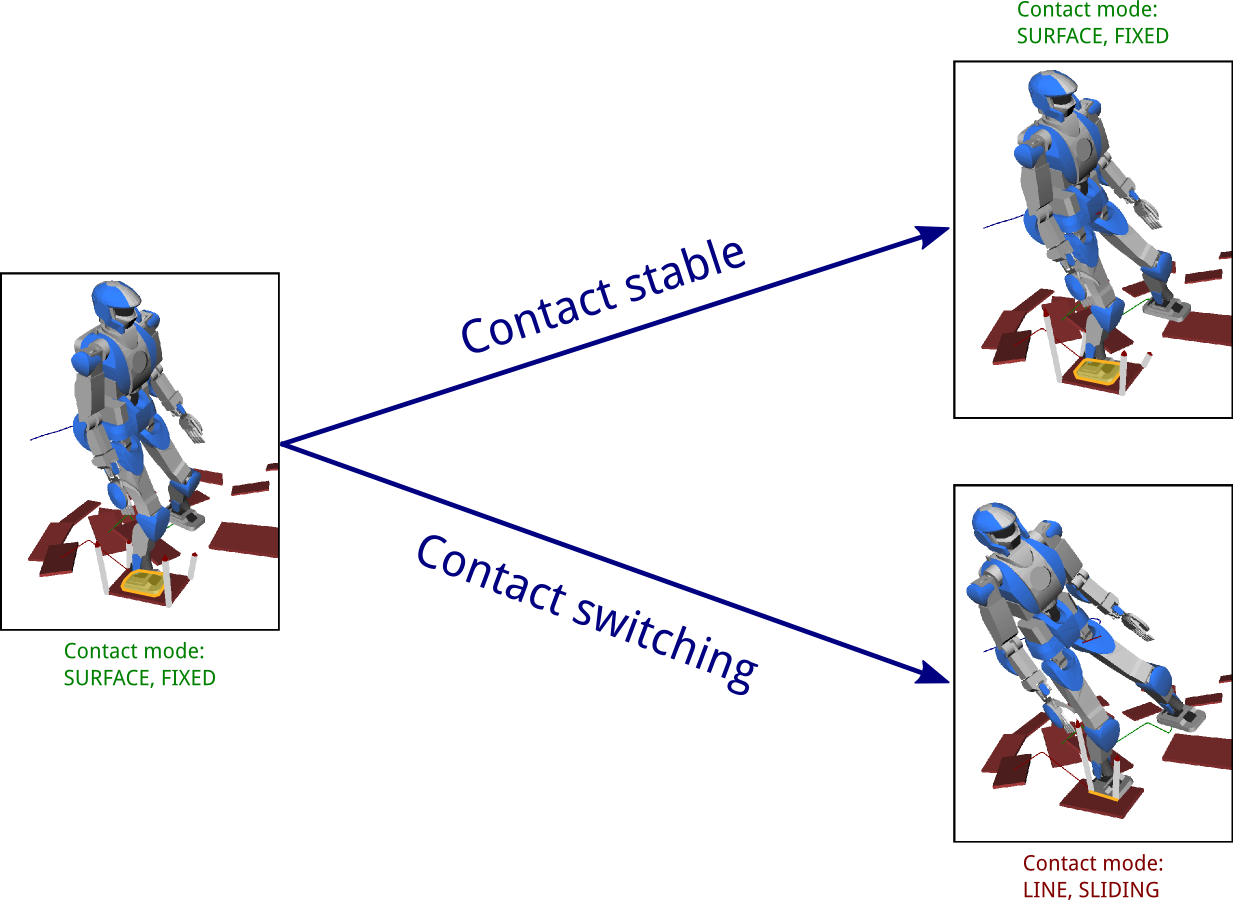## Contact stability of a motion

A motion q(t),τ(t),f(t),C(t) is a solution to the equations of motion:

M(q)¨q+h(q,˙q)=Sτ+iCJifi

A motion is contact stable if it contains no transition between contact modes, that is, if C(t) is stationary.

## Contact stability of a trajectory

A trajectory q(t) in contact state C is (weak) contact stable if it is possible to perform it with no contact switch:

{fiFCi},M(q)¨q+h(q,˙q)=Sτ+iCJifi

# Contact modes

## Complementarity

Let vi and fi denote the velocity and force on a given DOF i of a rigid body.

When one is equality-constrained, the other is always inequality-constrained:

 vi= vi≤ fi≤ fi=

## Contact mode

A contact mode specifies which of vi,fi is equality-constrained for all contact DOFs i.

## Example

In the fixed contact mode, all contact velocities are zero Ji˙q=0 and contact forces lie in their friction cones Fifi0.

# Contact mode graph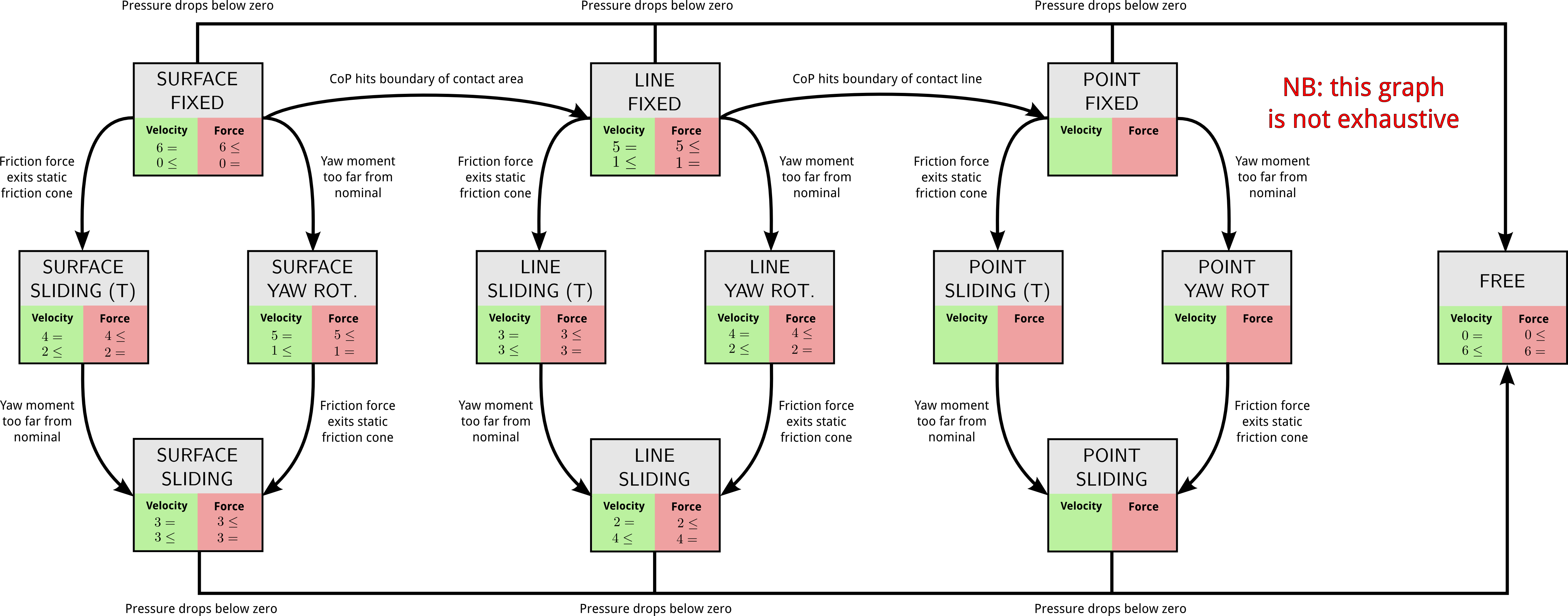# What we currently use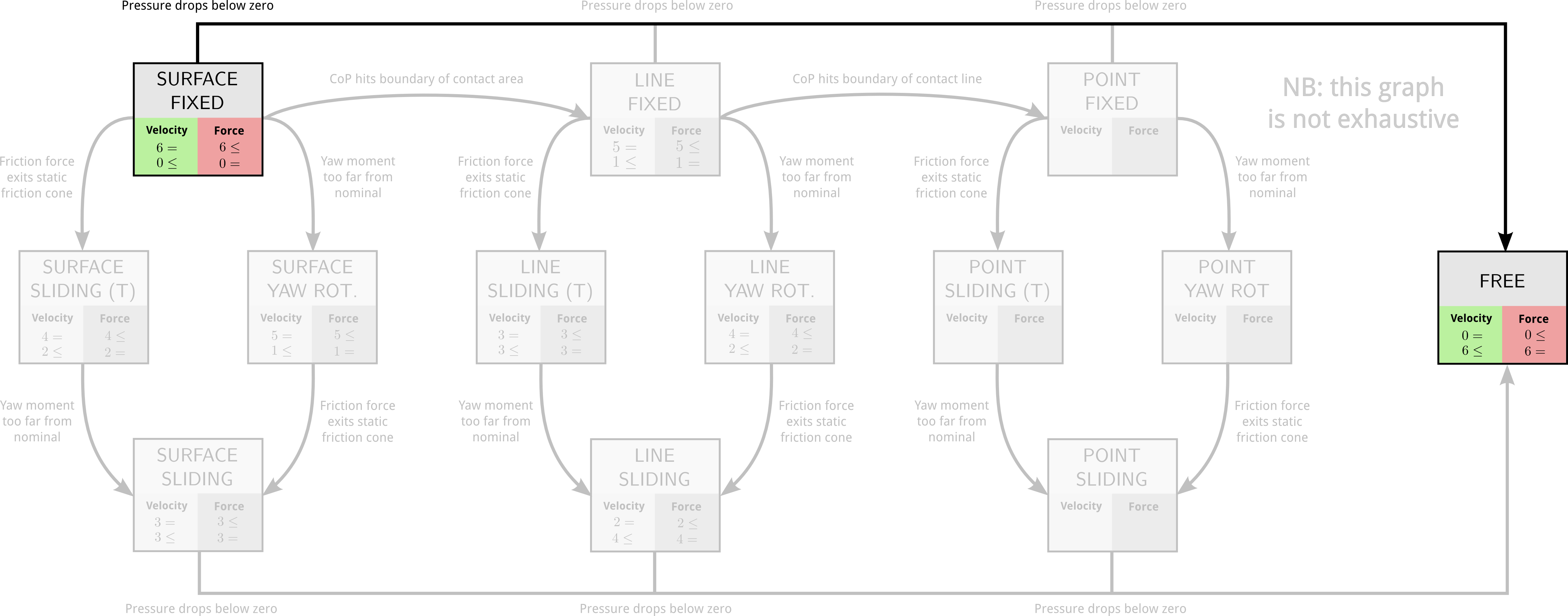# Single contact stability

in the fixed mode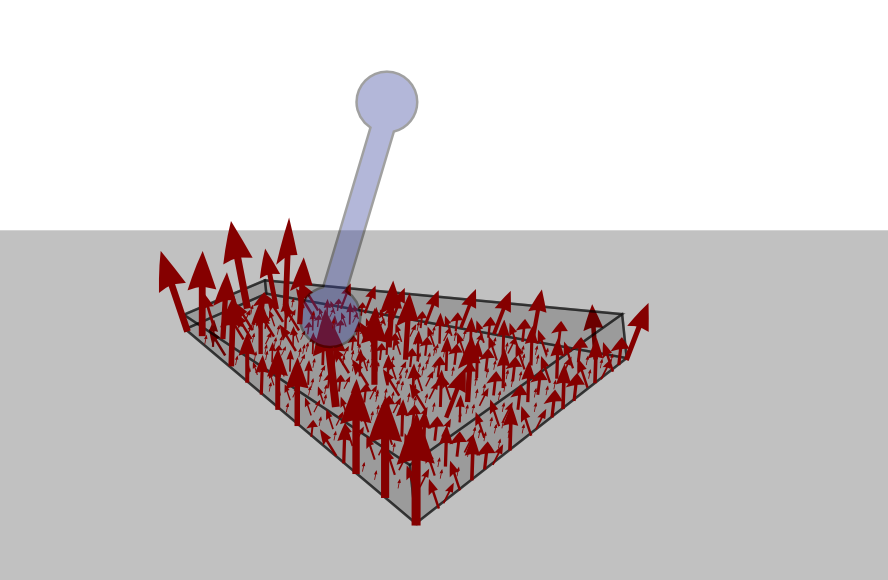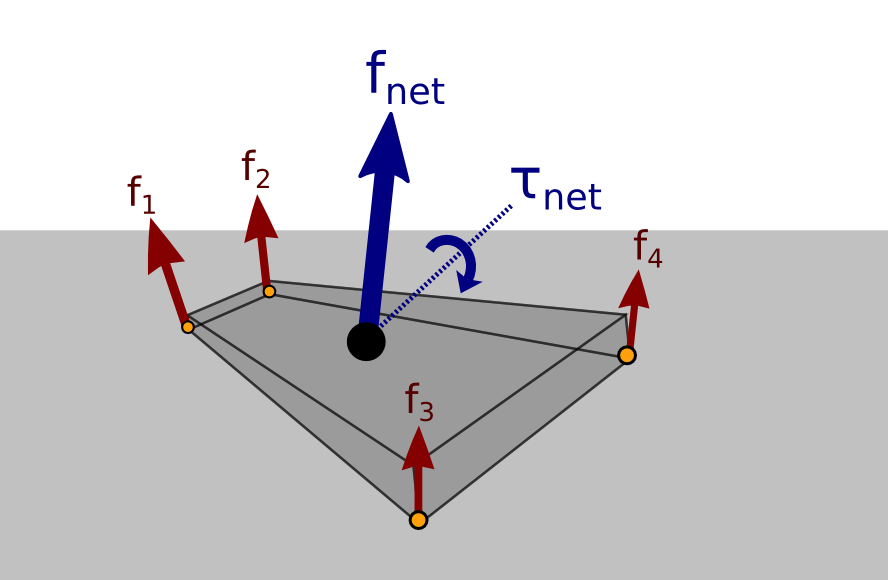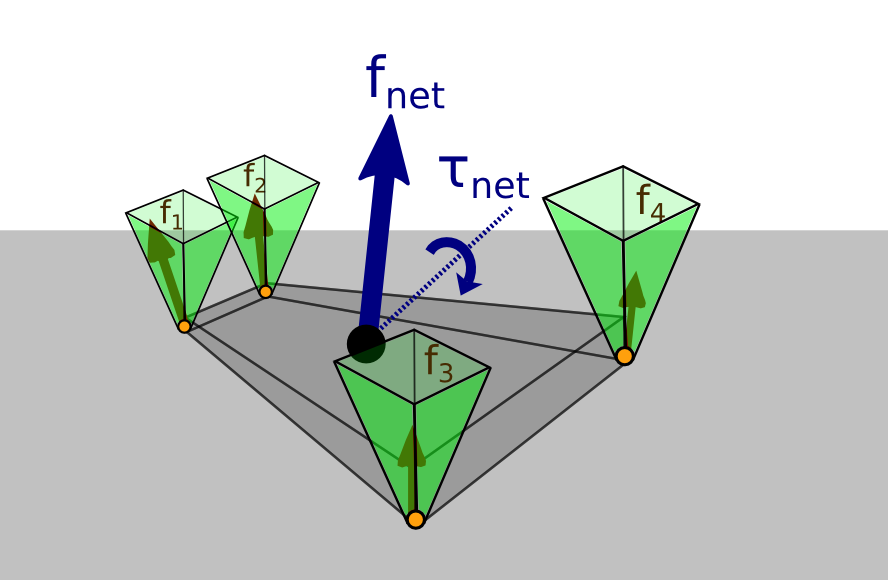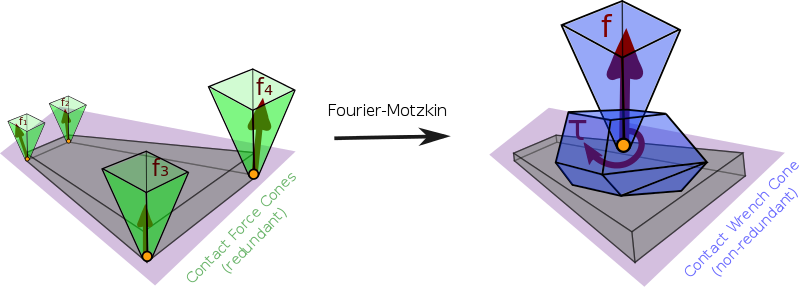## Contact stability

The Contact Wrench Cone characterizes contact stability, that is:

{fiFCi},M(q)¨q+h(q,˙q)=Sτ+4i=1JCifiwOCWCO,M(q)¨q+h(q,˙q)=Sτ+JOwO

## Structure of the CWC

• Friction cone on the net contact force
• Center of pressure condition
• Yaw moment condition

# Yaw moment condition

## “Torsional friction”

Model found in previous works:  |τzO|μτfz ... but physically incorrect.

## Condition found in the CWC

Taken at the center of pressure C, the correct condition is:

|τzCτsafe|  μdedgefz
• μ: usual static friction coefficient
• dedge: distance to the edge of the contact polygon
• τsafe: function of f and pC (details in (Caron, 2016), p.82)

# ZMP support areas

for multi-contact locomotion

# Zero-tilting Moment Point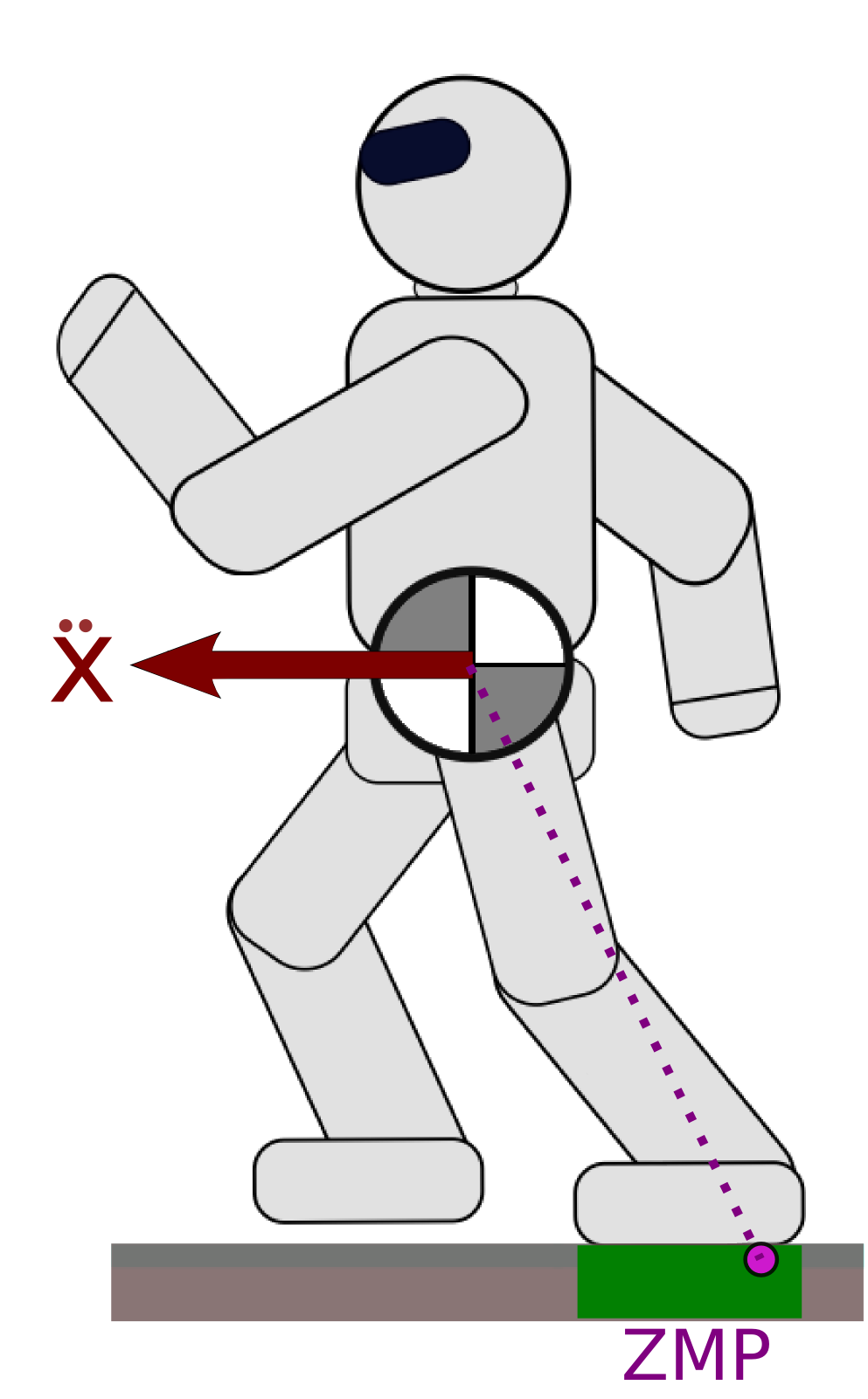## Definition

The ZMP is traditionally defined as the point on the floor where the moment of the contact wrench is parallel to the surface normal (Sardain & Bessonnet, 2004).

## Intuition as Center of Pressure

The point at which the robot “applies its weight”.

## Contact stability

The ZMP must lie inside the support polygon (convex hull of ground contact points).

# Limitations

• This definition requires a single “floor” surface (no multi-contact)
• The support polygon does not account for frictional limits (slippage, yaw rotations).

# ZMP of a Wrench

The ZMP is mathematically defined from a wrench (Sardain & Bessonnet, 2004). The ZMP in the plane Π(O,n) of normal n containing O is the point such that n×τZ=0, that is:

pZ=n×τOnf+pO.

We define the full support area S as the image of the CWC by this equation.

# Good news

This area can be computed geometrically (Caron et al., 2016):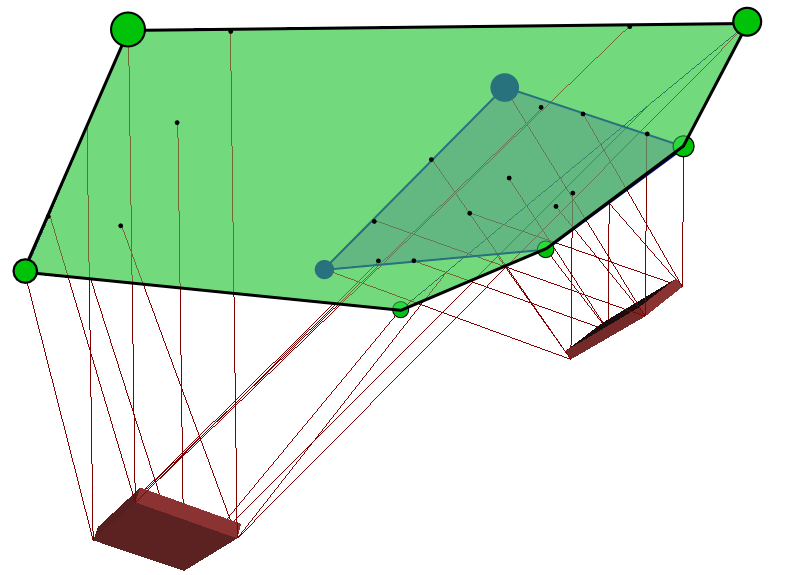It coincidates with the usual support area on horizontal floor.

The area is not always a polygon: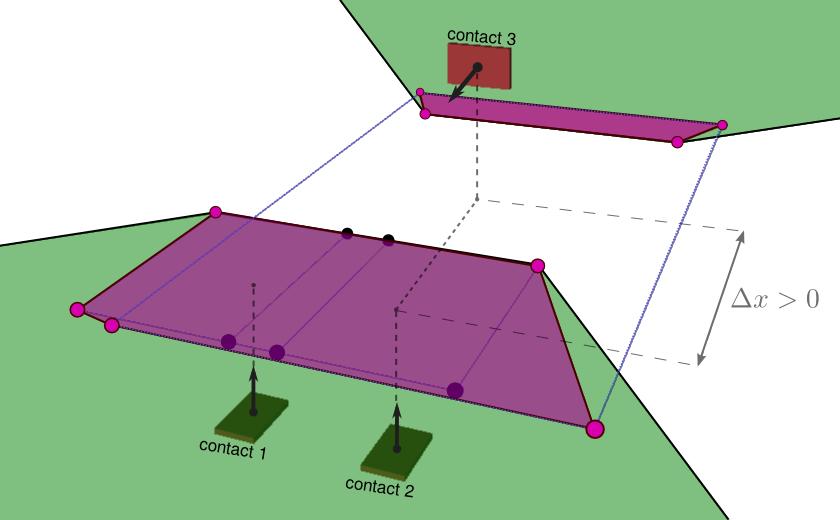This depends on the choice of the virtual plane normal n.

# Hands on!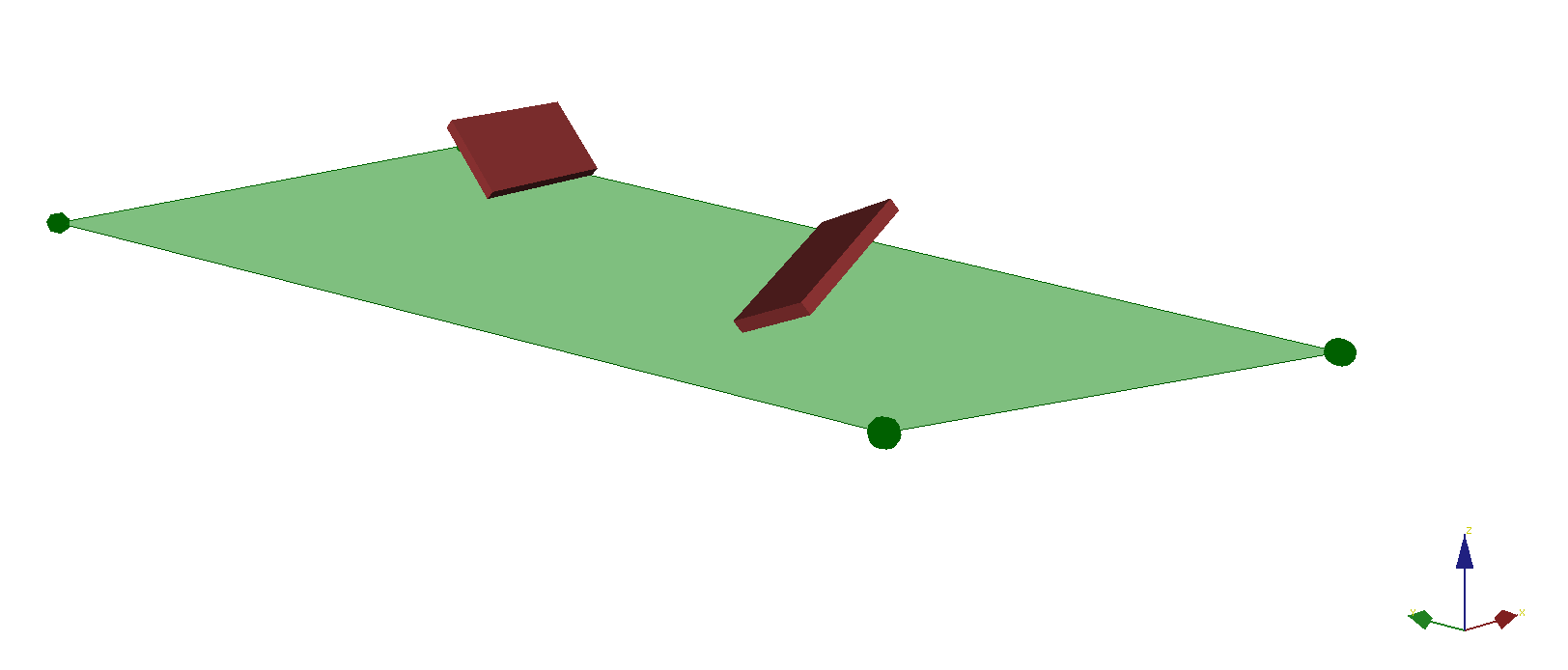# Decomposition

The equations of motion consist of two components:

• Newton-Euler equations: “external” interactions between environment and free-floating base:
[m¨pG˙LG]=[mg0]+contacti[fiGCi×fi]
• Actuated-joint equations: “internal” interactions between motors and joints:
M(q)¨q+h(q,˙q)=τ+iCJifi

# Newton-Euler equations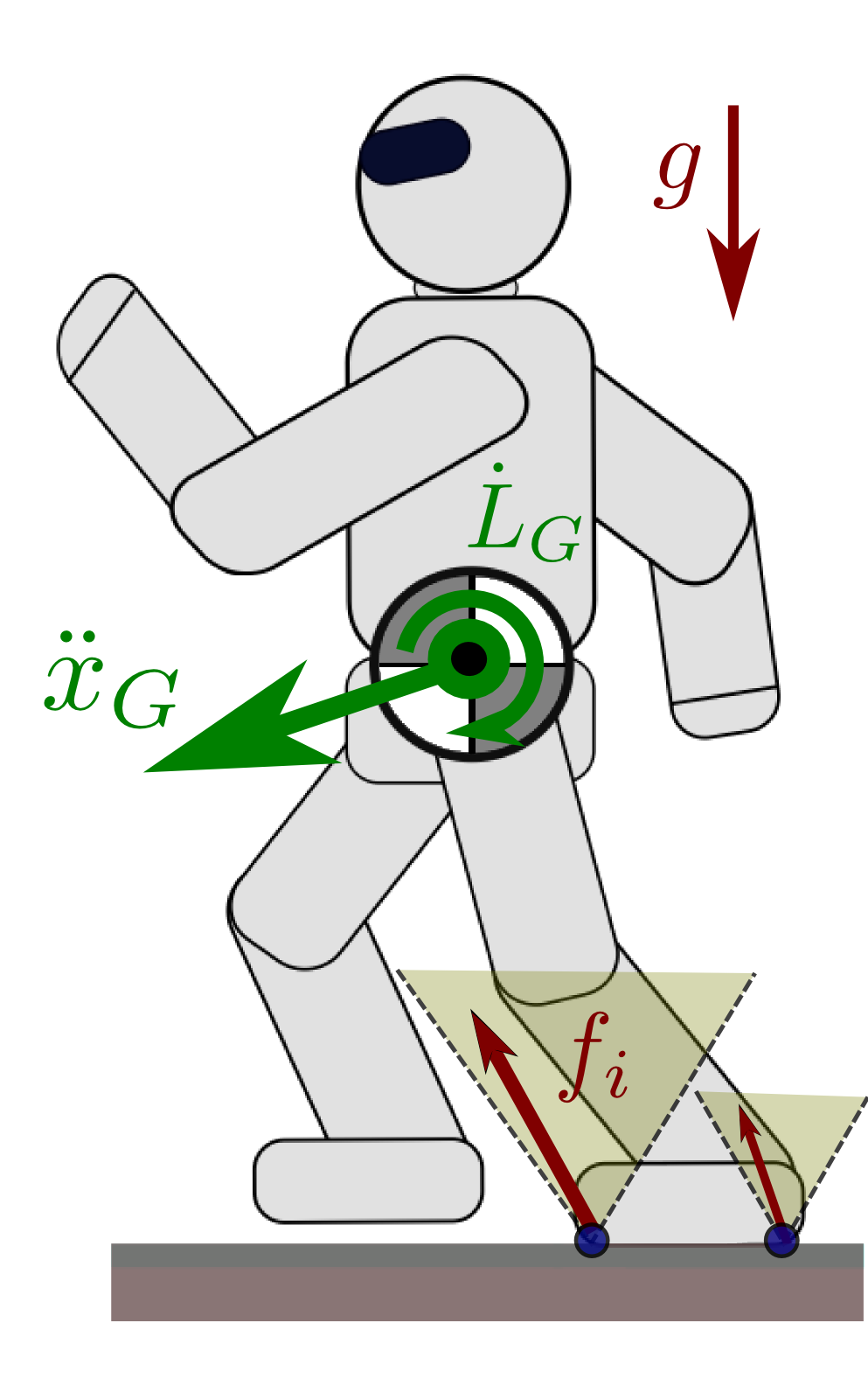[m¨pG˙LG]=[mg0]+contacti[fiGCi×fi]
• m and g: total mass and gravity vector
• ¨pG: acceleration of the center of mass (COM)
• ˙LG: rate of change of the angular momentum
• fi: contact force received at contact point Ci

## Hierarchical contact stability

First, solve contact stability for the COM and angular momentum trajectory.

Next, joint angles will follow...

# Linear Pendulum Mode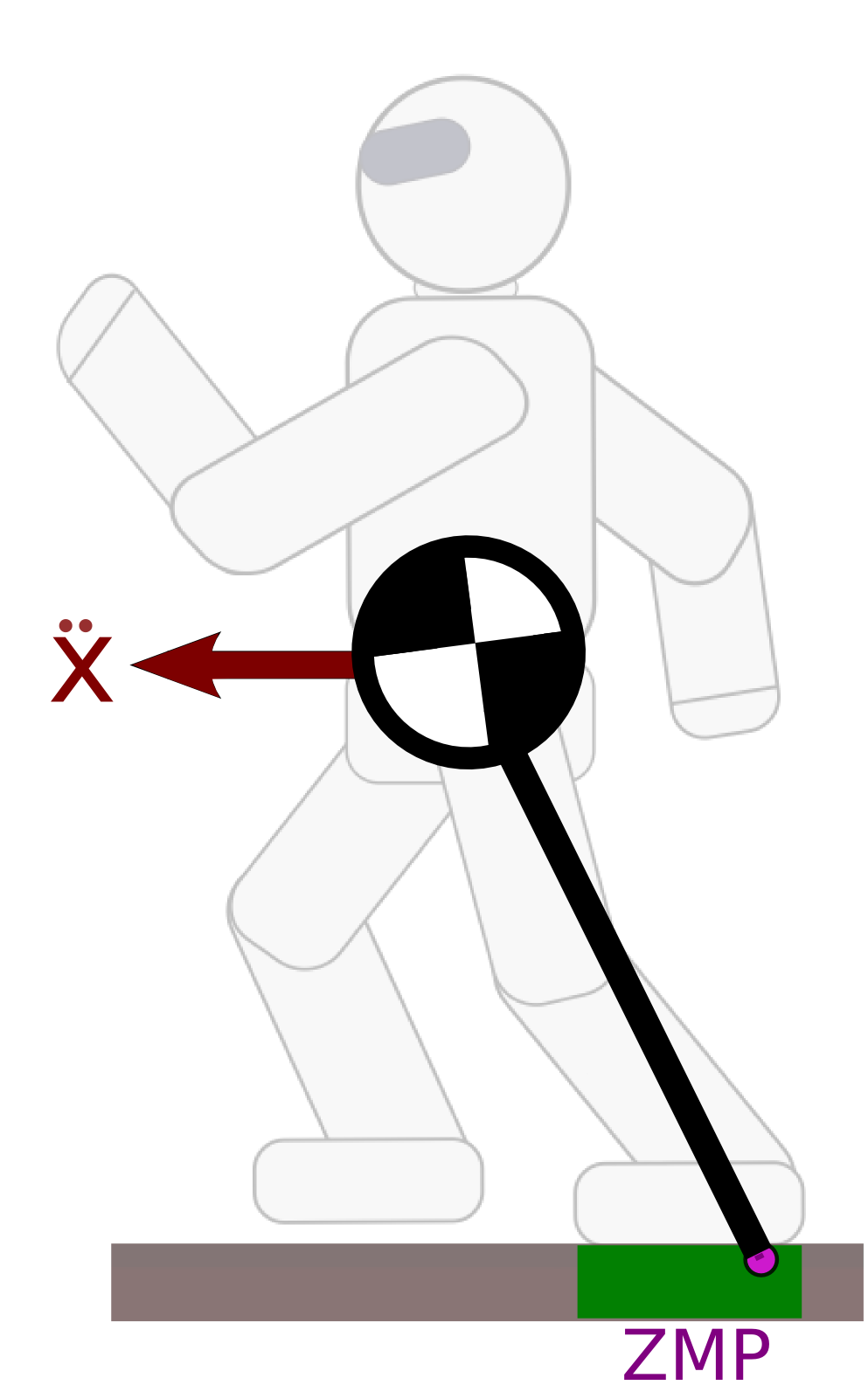The Newton equation can be written equivalently:

¨xG=g+¨zGzGzZ(xGxZ)˙LGxm(zGzZ)

and similarly for ¨yG. The Linear Inverted Pendulum Mode (Kajita et al., 2001) is obtained by constraining:

zGzZ=h˙LG=0

The system dynamics become ¨xG=gh(xZxG).

# Observation

The support area in the LIPM is smaller than the convex hull of contact points: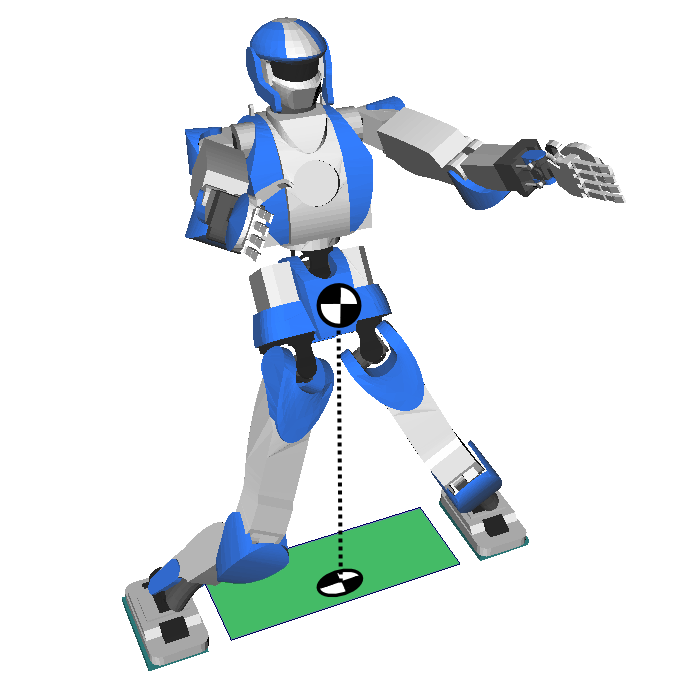We added 4 equality constraints to the resultant wrench, so the CWC is smaller.

# Pendular Support Area

The double-description method can be used (Caron et al., 2016) to compute the support area corresponding to the system:

wCWCzGzZ=h˙LG=0

We call it the pendular support area.

It is smaller than the full support area and characterizes contact stability in the pendular mode.

# Linear Pendulum Mode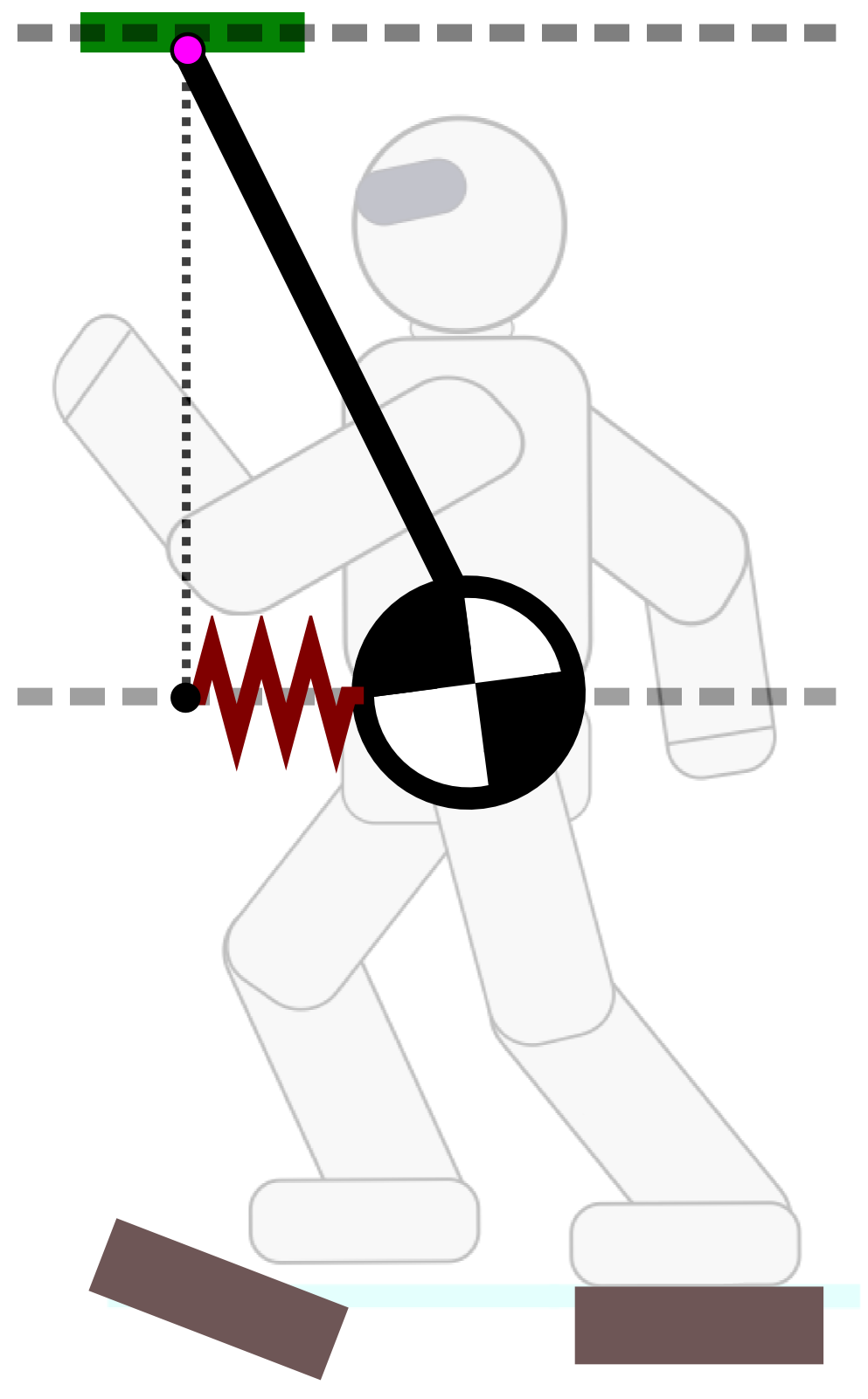Let us consider the ZMP above the COM:

¨xG = gh(xGxZ)

This is the dynamic equation of a spring.

## Attractivity

• LIPM: the ZMP is a repellor of the COM
• LPM: the ZMP is a marginal attractor of the COM

Control law where the robot is driven from above.

# Demo 2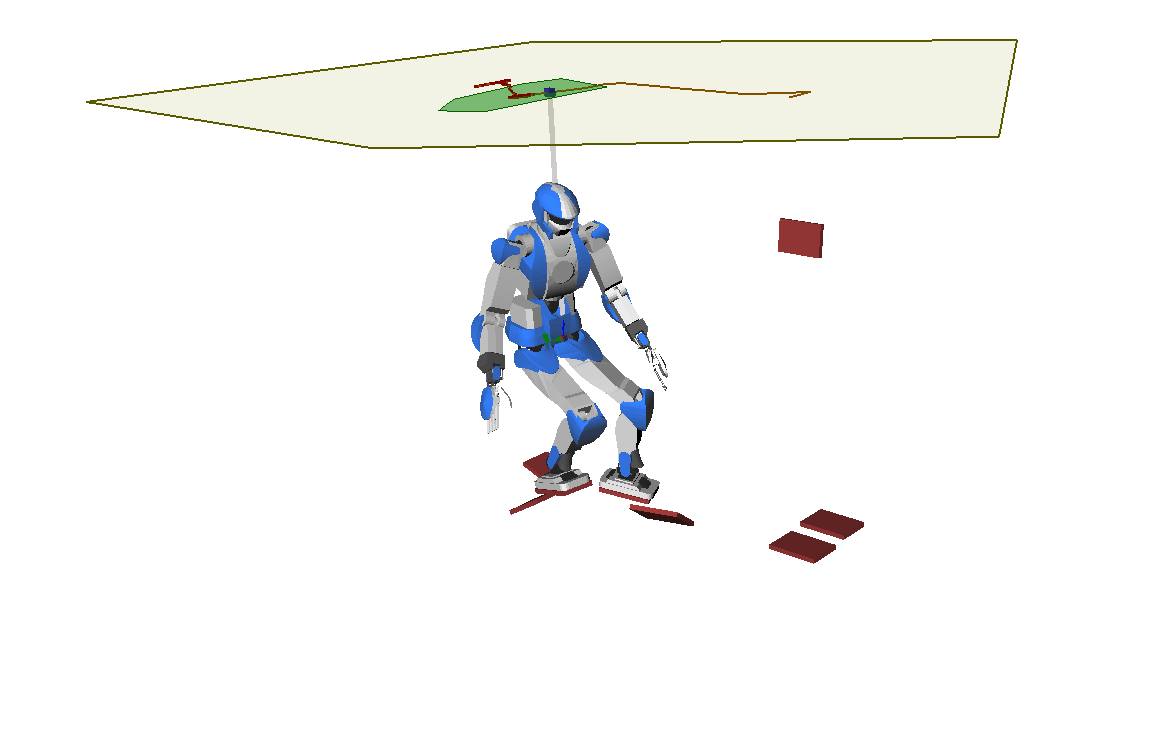# Beyond Good and ZMP

a multi-contact walking pattern generator

# Cone of 3D COM Accelerations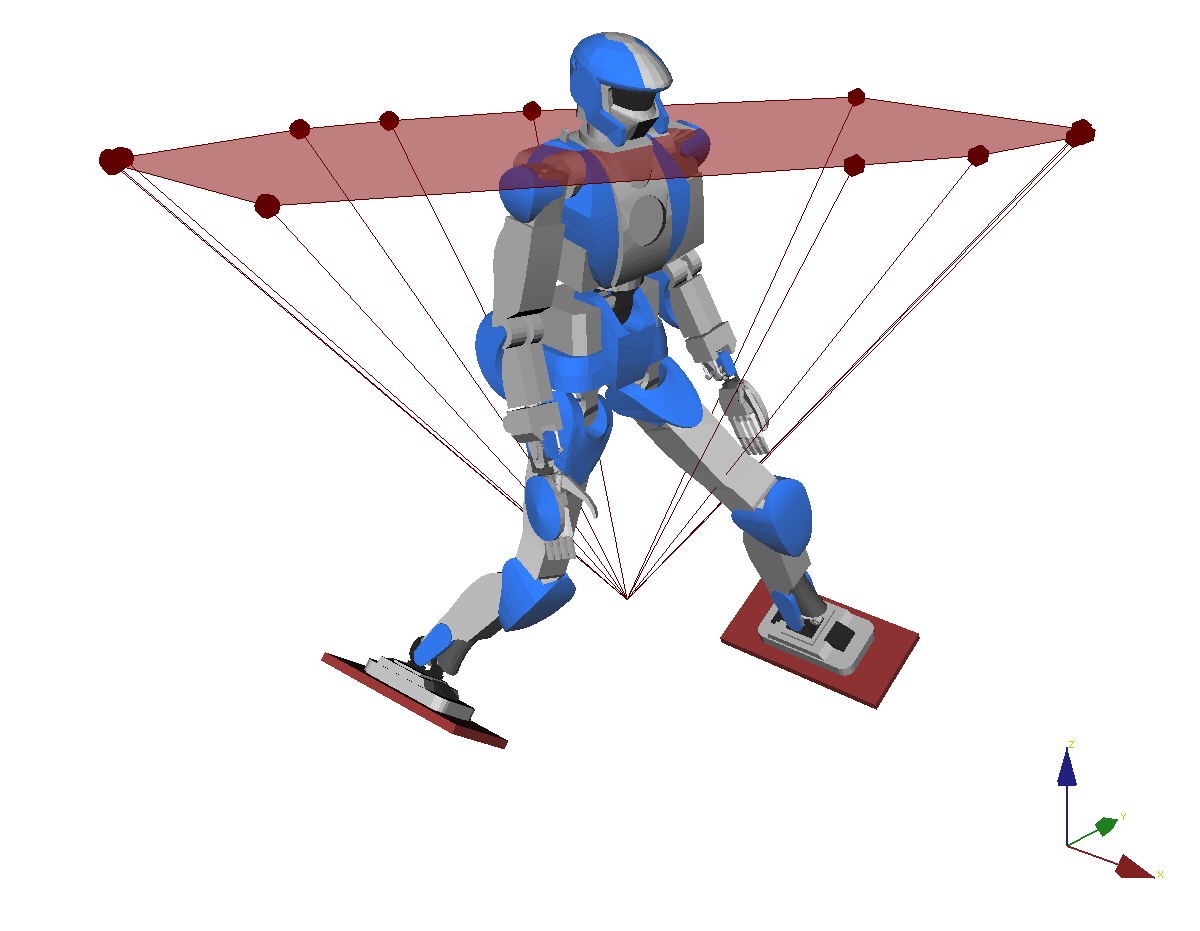## Beyond ZMP

Removes the planar COM constraint.

## Calculation

Algorithm to compute it as convex hull of 2D dual points, fast and numerically robust (Caron & Kheddar, submitted).

# Predictive control

Consider the linear control problem:

x(k+1)=Ax(k)+Bu(k)
• x(k): COM state (position and velocity) at preview time kΔT
• u(k): COM acceleration at preview time kΔT

## Optimization problem

Predictive control achieves a task over a preview horizon:

min{u(k)} x(N)xgoal2+ϵku(k)2s.t. k, u(k)C(x(k))

where C(x(k)) is the COM acceleration cone at time step k. This constraint is linear-quadratic due to its dependency on x(k).

# Linearizing the MPC problem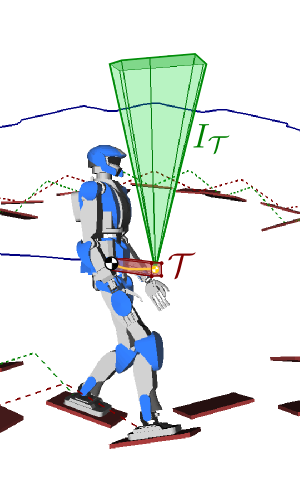## Idea

Bound the preview COM trajectory in a tube T, then compute the intersection:

IT=pGTC(pG)

Conservative contact-stability criterion.

## Inequality constraints

Decouples u(k)C(x(k)) into:

CTu(k)0PTx(k)0

# Demo 3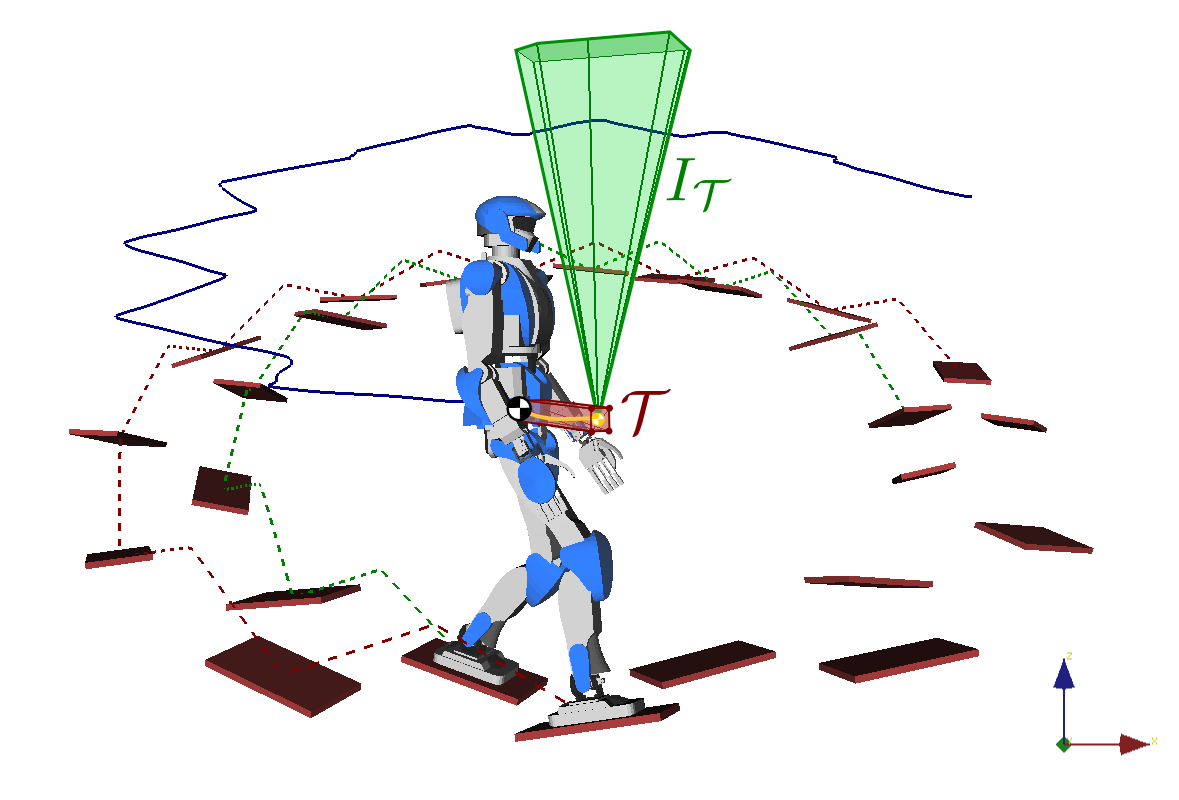# When to make a step?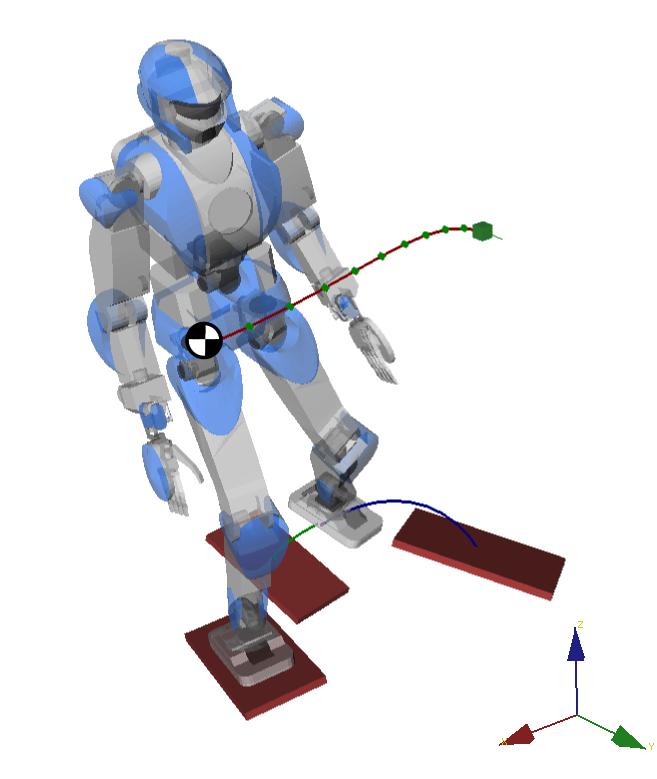Problem: fixed step timings do not work any more in multi-contact (now depends on terrain topology).

## Time-Optimal Path Parameterization

Given a path q(s) and dynamic constraints, TOPP finds a retiming q(t) satisfying all constraints and with minimum duration.

## Integration with the MPC

Continuous interpolation/retiming loop running at 20-30 Hz thanks to a new TOPP-Polygon algorithm.

# Demo 4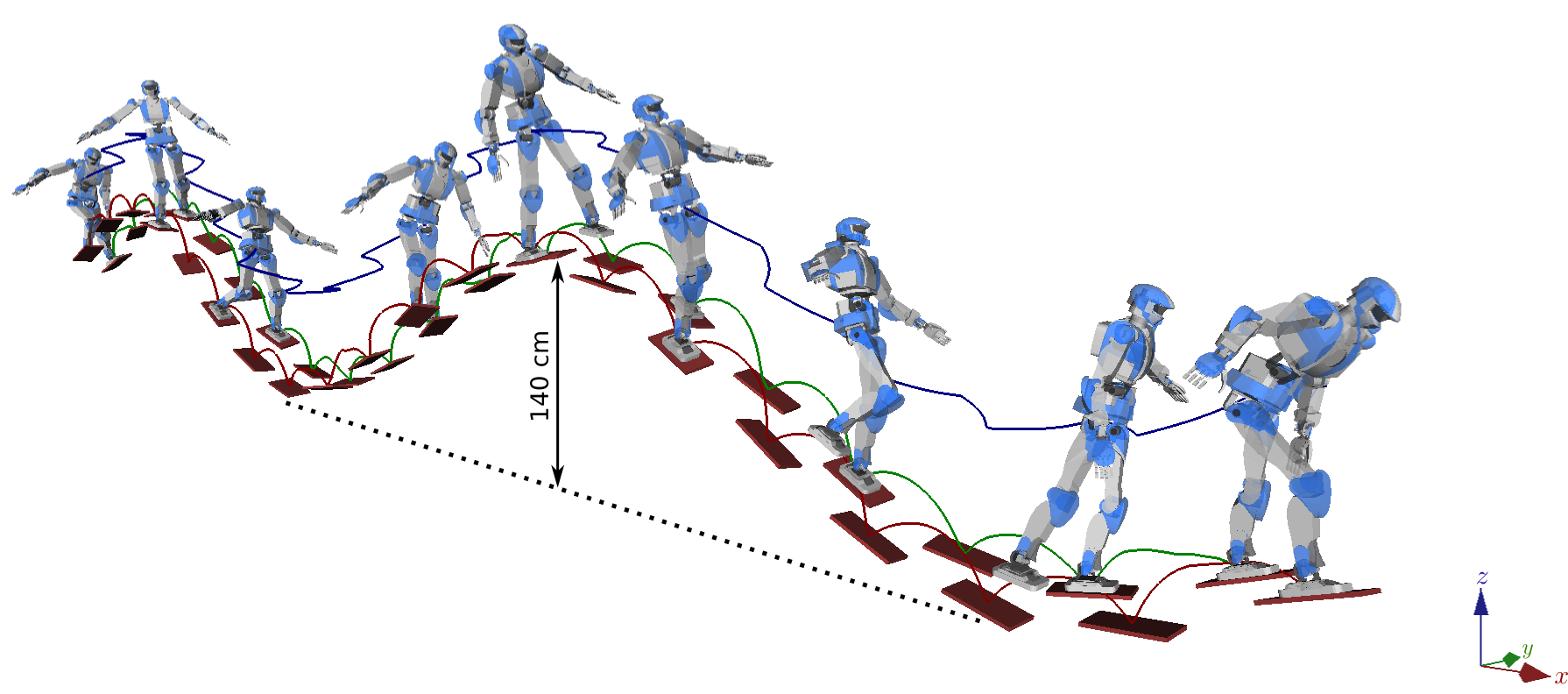# Wrapping remarks

## Single contact stability

Analytical formula of the CWC: friction cone, COP area, yaw moment.

## ZMP support areas

Applicable to general multi-contact locomotion, but COM planar constraint.

## 3D COM acceleration cones

Generalizes the latter. Computed fast enough for the control loop.

## Multi-contact Walking Pattern Generator

Use a QCQP solver, or... linearize inequality constraints.